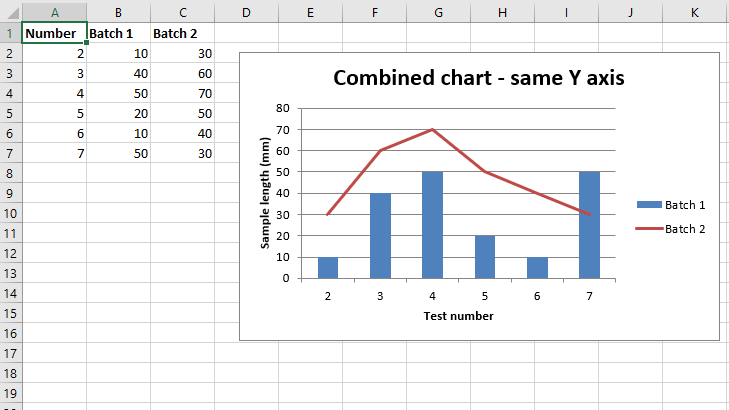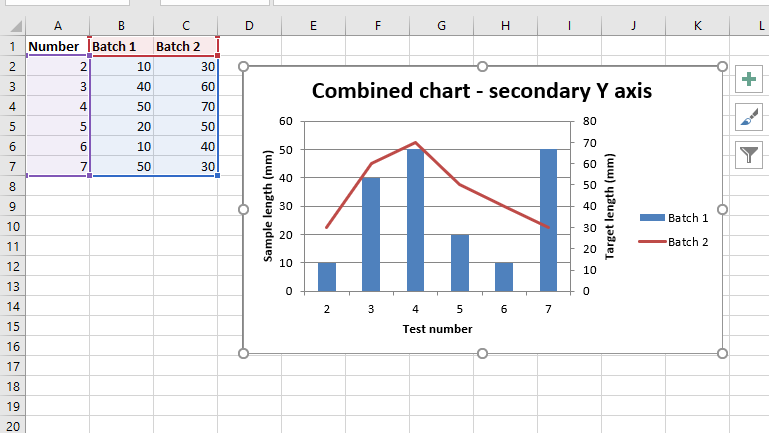Open in App
Not now

# Python | Plotting Combined charts in excel sheet using XlsxWriter module

• Difficulty Level : Medium
• Last Updated : 30 Jan, 2022

Prerequisite: Create and Write on an excel sheet
XlsxWriter is a Python library using which one can perform multiple operations on excel files like creating, writing, arithmetic operations, and plotting graphs. Let’s see how to plot Combined charts using real-time data.
Charts are composed of at least one series of one or more data points. Series themselves are comprised of references to cell ranges. For plotting the charts on an excel sheet, firstly, create a chart object of specific chart types ( i.e Line, Column chart, etc.). After creating chart objects, insert data in it, and lastly, add that chart object in the sheet object.

Code #1: Plot a combined column and line chart that shares the same X and Y axes.
For plotting the Combined chart on an excel sheet, use the combine() method of the chart object for combining Two chart objects together.

## Python3

 `# import xlsxwriter module``import` `xlsxwriter``  ` `# Workbook() takes one, non-optional, argument  ``# which is the filename that we want to create.``workbook ``=` `xlsxwriter.Workbook(``'chart_combined.xlsx'``)` `# The workbook object is then used to add new  ``# worksheet via the add_worksheet() method.``worksheet ``=` `workbook.add_worksheet()` `# Create a new Format object to formats cells``# in worksheets using add_format() method .``  ` `# here we create bold format object .``bold ``=` `workbook.add_format({``'bold'``: ``True``})` `# Add the worksheet data that the charts will refer to.``headings ``=` `[``'Number'``, ``'Batch 1'``, ``'Batch 2'``]``data ``=` `[``    ``[``2``, ``3``, ``4``, ``5``, ``6``, ``7``],``    ``[``10``, ``40``, ``50``, ``20``, ``10``, ``50``],``    ``[``30``, ``60``, ``70``, ``50``, ``40``, ``30``],``]` `# Write a row of data starting from 'A1'``# with bold format .``worksheet.write_row(``'A1'``, headings, bold)` `# Write a column of data starting from ``# 'A2', 'B2', 'C2' respectively .``worksheet.write_column(``'A2'``, data[``0``])``worksheet.write_column(``'B2'``, data[``1``])``worksheet.write_column(``'C2'``, data[``2``])` `# Create a chart object that can be added``# to a worksheet using add_chart() method.` `# here we create a column chart object .``# This will use as the primary chart.``column_chart1 ``=` `workbook.add_chart({``'type'``: ``'column'``})` `# Add a data series to a chart``# using add_series method.``  ` `# Configure the first series.``# = Sheet1 !\$A\$1 is equivalent to ['Sheet1', 0, 0].` `# note : spaces is not inserted in b / w``# = and Sheet1, Sheet1 and !``# if space is inserted it throws warning.``column_chart1.add_series({``    ``'name'``:       ``'= Sheet1 !\$B\$1'``,``    ``'categories'``: ``'= Sheet1 !\$A\$2:\$A\$7'``,``    ``'values'``:     ``'= Sheet1 !\$B\$2:\$B\$7'``,``})` `# Create a new line chart.``# This will use as the secondary chart.``line_chart1 ``=` `workbook.add_chart({``'type'``: ``'line'``})` `# Configure the data series for the secondary chart.``line_chart1.add_series({``    ``'name'``:       ``'= Sheet1 !\$C\$1'``,``    ``'categories'``: ``'= Sheet1 !\$A\$2:\$A\$7'``,``    ``'values'``:     ``'= Sheet1 !\$C\$2:\$C\$7'``,``})` `# Combine both column and line charts together.``column_chart1.combine(line_chart1)` `# Add a chart title ``column_chart1.set_title({ ``'name'``: ``'Combined chart - same Y axis'``})` `# Add x-axis label``column_chart1.set_x_axis({``'name'``: ``'Test number'``})` `# Add y-axis label``column_chart1.set_y_axis({``'name'``: ``'Sample length (mm)'``})` `# add chart to the worksheet with given``# offset values at the top-left corner of``# a chart is anchored to cell D2``worksheet.insert_chart(``'D2'``, column_chart1, {``'x_offset'``: ``25``, ``'y_offset'``: ``10``})` `# Finally, close the Excel file ``# via the close() method. ``workbook.close()`

Output :Code #2: Plot a combined column and line chart in which the secondary chart will have a secondary Y-axis.
For plotting the Combined chart with the secondary Y-axis on an excel sheet, We set a secondary Y-axis via ‘y2_axis’. keyword argument of the add_series() method of the respective chart object.

## Python3

 `# import xlsxwriter module``import` `xlsxwriter``  ` `# Workbook() takes one, non-optional, argument  ``# which is the filename that we want to create.``workbook ``=` `xlsxwriter.Workbook(``'combined_chart_secondaryAxis.xlsx'``)` `# The workbook object is then used to add new  ``# worksheet via the add_worksheet() method.``worksheet ``=` `workbook.add_worksheet()` `# Create a new Format object to formats cells``# in worksheets using add_format() method .``  ` `# here we create bold format object .``bold ``=` `workbook.add_format({``'bold'``: ``True``})` `# Add the worksheet data that the charts will refer to.``headings ``=` `[``'Number'``, ``'Batch 1'``, ``'Batch 2'``]``data ``=` `[``    ``[``2``, ``3``, ``4``, ``5``, ``6``, ``7``],``    ``[``10``, ``40``, ``50``, ``20``, ``10``, ``50``],``    ``[``30``, ``60``, ``70``, ``50``, ``40``, ``30``],``]` `# Write a row of data starting from 'A1'``# with bold format .``worksheet.write_row(``'A1'``, headings, bold)` `# Write a column of data starting from ``# 'A2', 'B2', 'C2' respectively .``worksheet.write_column(``'A2'``, data[``0``])``worksheet.write_column(``'B2'``, data[``1``])``worksheet.write_column(``'C2'``, data[``2``])` `# Create a chart object that can be added``# to a worksheet using add_chart() method.` `# here we create a column chart object .``# This will use as the primary chart.``column_chart2 ``=` `workbook.add_chart({``'type'``: ``'column'``})` `# Add a data series to a chart``# using add_series method.``  ` `# Configure the first series.``# = Sheet1 !\$A\$1 is equivalent to ['Sheet1', 0, 0].` `# note : spaces is not inserted in b / w``# = and Sheet1, Sheet1 and !``# if space is inserted it throws warning.``column_chart2.add_series({``    ``'name'``:       ``'= Sheet1 !\$B\$1'``,``    ``'categories'``: ``'= Sheet1 !\$A\$2:\$A\$7'``,``    ``'values'``:     ``'= Sheet1 !\$B\$2:\$B\$7'``,``})` `# Create a new line chart.``# This will use as the secondary chart.``line_chart2 ``=` `workbook.add_chart({``'type'``: ``'line'``})` `# Configure the data series for the secondary chart.``# We also set a secondary Y axis via (y2_axis).``line_chart2.add_series({``    ``'name'``:       ``'= Sheet1 !\$C\$1'``,``    ``'categories'``: ``'= Sheet1 !\$A\$2:\$A\$7'``,``    ``'values'``:     ``'= Sheet1 !\$C\$2:\$C\$7'``,``     ``'y2_axis'``:    ``True``,``})` `# Combine both column and line charts together.``column_chart2.combine(line_chart2)` `# Add a chart title ``column_chart2.set_title({ ``'name'``: ``'Combined chart - secondary Y axis'``})` `# Add x-axis label``column_chart2.set_x_axis({``'name'``: ``'Test number'``})` `# Add y-axis label``column_chart2.set_y_axis({``'name'``: ``'Sample length (mm)'``})` `# Note: the y2 properties are on the secondary chart.``line_chart2.set_y2_axis({``'name'``: ``'Target length (mm)'``})` `# add chart to the worksheet with given``# offset values at the top-left corner of``# a chart is anchored to cell D2``worksheet.insert_chart(``'D2'``, column_chart2, {``'x_offset'``: ``25``, ``'y_offset'``: ``10``})` `# Finally, close the Excel file ``# via the close() method. ``workbook.close()`

Output :My Personal Notes arrow_drop_up